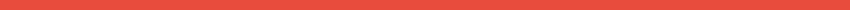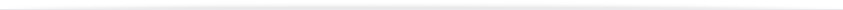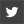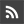Monetary LibraryNEWSAbout UsContact UsWebsiteBizcommunity≡NEWS# How to improve investing strategies with the use of Excel

The Excel spreadsheet program from Microsoft is a really important tool these days for investors. They use it in order to track the activity of the investments in a manner that is completely organised. You can so easily use features like the drop-down list in Excel to track investment positions and even for risk management purposes.
Tracking investments with Microsoft Excel

You can use Excel spreadsheets in many different ways to track activity of investments. Everything starts with figuring out what data is to be included. In most cases the investors track entry, date, sizes and closing prices. Differences between entry prices and closing prices are normal, just as percentage returns, standard deviation, losses and profits over given periods. Adding different data that is to be analysed will be very simple but the huge advantage appears in automatically calculating different statistics of importance for the investors. Normally one Excel workbook is utilised for one stock.

Creating calculation formulas

This is where Excel shines. Those formulas that are normally used are the following:
• Differences – For instance, calculating the difference between entry price and current stock price.
• Percent return – This is basically the difference between the price of the moment and the entry price, divided with the entry price. It is a really simple formula to set up in Excel.
• Profits and losses – You take the profit and loss difference and you multiply it by shares number.
• Standard deviation – Measuring portfolio’s volatility. You can use Excel to calculate deviation for different data sets with only a few keystrokes.
Portfolio standard deviation calculation

The user can easily compile data stored in the individual Excel sheets in order to determine various metrics like overall standard deviation and the overall profit. Calculating based on the classic factors taken into account is quite simple. If the user is versatile in Excel the data that is calculated from one sheet can actually be calculated and shown in another sheet, one that would only highlight profits and losses or standard deviation for all the stocks.

Importing data

Microsoft Excel is nowadays really popular in basically all industries that see the use of a computer to analyse any type of data. Because of this, it becomes really easy to receive the data that is offered by an analysis tool and then import it into the sheets used by the investor. Any data export and import is possible with only a few clicks. That is definitely something that helps a lot at the end of the day as it makes analysis a lot faster.

Final thoughts

On the whole, using Microsoft Excel to assist with investment evaluation and organization is definitely something that will help out a lot at the end of the day. The one part of the process that lasts the longest time is formatting the sheet. However, after this phase is over the only thing that has to be done is importing new data or adding it manually. Calculations are done automatically and the important analysis can be done quite fast.

16 Aug 2016 11:37

<<Back